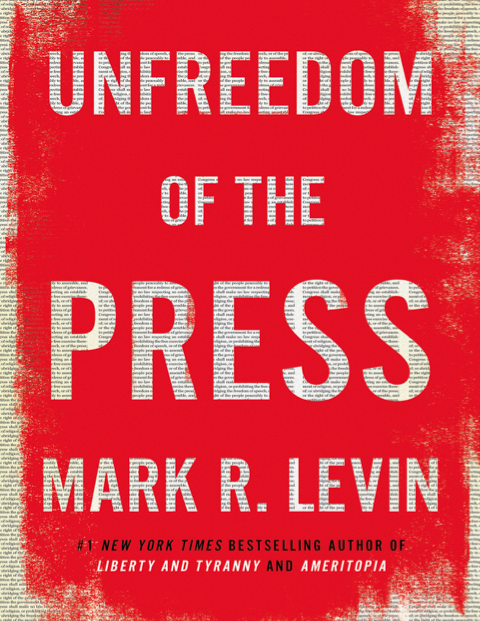۰
سبد خریدهر روز با کتاب‌های بیشتر# Unfreedom of the Press | Mark R. Levin

کد محصول: eSHB-1144
۱۱,۶۹۰ تومانافزودن به سبد خرید
• درباره کتاب
• بخشی از کتاب
• نظرات
•• ## تخفیف ویژه | اولین سفارش

<% if (product.thumbnail) { %><% } %>

### <%- product.title %>

<% if (Array.isArray(product.attributes)) { %>
<% _.forEach(product.attributes, function(attribute, index) { %>
• <%- attribute.name %>: <%- attribute.value %>
• <% }); %>
<% } %><% if (product.price_label) { %>
<%- (product.price_label.toString()) %>
<% } else { %><% if (product.in_stock == 1) { %>
<% if (product.sale_price) { %><%- (product.price.toString().formatNumber().convertToLocalNumber() + currency_sign) %><%- (product.sale_price.toString().formatNumber().convertToLocalNumber() + currency_sign) %><% } else { %><%- (product.price.toString().formatNumber().convertToLocalNumber() + currency_sign) %><% } %>
<% } else { %>
اتمام موجودی
<% } %><% } %>
<% if (product.ribbon) { %>
<%- product.ribbon %>
<% } %><% if (product.sale_amount) { %><% if (product.in_stock==1) { %>
<% if (product.sale_type==2) { %> <%- ((product.sale_amount).toString().formatNumber().convertToLocalNumber() + currency_sign) %><% } else { %> <%- (product.sale_amount.toString().formatNumber().convertToLocalNumber()) %> درصد <% } %>
<% } %><% } %>رمز عبورتان را فراموش کرده‌اید؟

ثبت کلمه عبور خود را فراموش کرده‌اید؟ لطفا شماره همراه یا آدرس ایمیل خودتان را وارد کنید. شما به زودی یک ایمیل یا اس ام اس برای ایجاد کلمه عبور جدید، دریافت خواهید کرد.

بازگشت به بخش ورود

کد دریافتی را وارد نمایید.

بازگشت به بخش ورود

### مشاهده سفارش

<%- order.customer_name.toString() %>
<%- order.id.toString().convertToLocalNumber() %>
<%- order.customer_province.toString() %>-<%- order.customer_city.toString() %>-<%- order.customer_address.toString() %>
<%- order.customer_mobile.toString().convertToLocalNumber() %>
<%- order.shipping_name.toString() %>
<%- (Number(order.total_shipping).toString().formatNumber().convertToLocalNumber() + currency_sign) %>
<%- order.payment_method_name.toString() %>
<%- (Number(order.total).toString().formatNumber().convertToLocalNumber() + currency_sign) %>
<% if(order.tracking_number) { %>
<%- order.tracking_number.toString() %>
<% } %>
نام محصول
تعداد
قیمت واحد
قیمت کل
تخفیف
قیمت نهایی
<% \$.each(products, function(index,product) { %><%- product.name %>
<%- product.quantity %>
<%- (Number(product.original_price).toString().formatNumber().convertToLocalNumber() + currency_sign) %>
<%- (Number(product.original_price*product.quantity).toString().formatNumber().convertToLocalNumber() + currency_sign) %>
<%- (Number(product.discount).toString().formatNumber().convertToLocalNumber() + currency_sign) %>
<%- (Number(product.total).toString().formatNumber().convertToLocalNumber() + currency_sign) %>
<% }); %>
<% if(!orders.length) { %>

شما هنوز هیچ سفارشی ثبت نکرده‌اید.

<% } else { %>
• شماره سفارش
تاریخ سفارش
پرداخت
وضعیت
جمع نهایی
• <% \$.each(orders, function(index,order) { %>
• <%- order.id.toString().convertToLocalNumber() %>